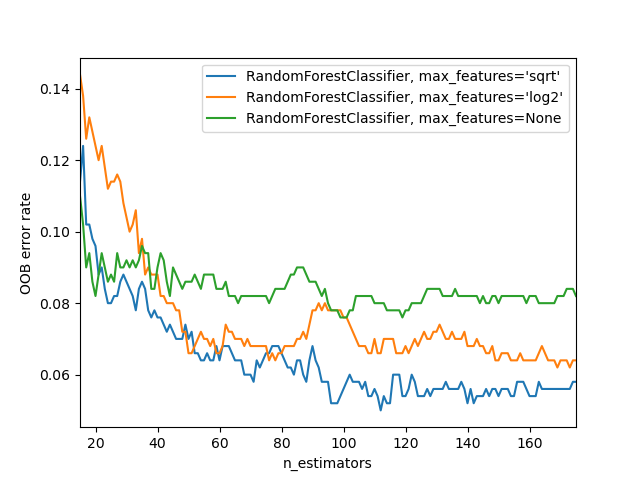# OOB Errors for Random Forests¶

The RandomForestClassifier is trained using bootstrap aggregation, where each new tree is fit from a bootstrap sample of the training observations $$z_i = (x_i, y_i)$$. The out-of-bag (OOB) error is the average error for each $$z_i$$ calculated using predictions from the trees that do not contain $$z_i$$ in their respective bootstrap sample. This allows the RandomForestClassifier to be fit and validated whilst being trained 1.

The example below demonstrates how the OOB error can be measured at the addition of each new tree during training. The resulting plot allows a practitioner to approximate a suitable value of n_estimators at which the error stabilizes.

1

T. Hastie, R. Tibshirani and J. Friedman, “Elements of Statistical Learning Ed. 2”, p592-593, Springer, 2009.import matplotlib.pyplot as plt

from collections import OrderedDict
from sklearn.datasets import make_classification
from sklearn.ensemble import RandomForestClassifier

# Author: Kian Ho <hui.kian.ho@gmail.com>
#         Gilles Louppe <g.louppe@gmail.com>
#         Andreas Mueller <amueller@ais.uni-bonn.de>
#

print(__doc__)

RANDOM_STATE = 123

# Generate a binary classification dataset.
X, y = make_classification(n_samples=500, n_features=25,
n_clusters_per_class=1, n_informative=15,
random_state=RANDOM_STATE)

# NOTE: Setting the warm_start construction parameter to True disables
# support for parallelized ensembles but is necessary for tracking the OOB
# error trajectory during training.
ensemble_clfs = [
("RandomForestClassifier, max_features='sqrt'",
RandomForestClassifier(warm_start=True, oob_score=True,
max_features="sqrt",
random_state=RANDOM_STATE)),
("RandomForestClassifier, max_features='log2'",
RandomForestClassifier(warm_start=True, max_features='log2',
oob_score=True,
random_state=RANDOM_STATE)),
("RandomForestClassifier, max_features=None",
RandomForestClassifier(warm_start=True, max_features=None,
oob_score=True,
random_state=RANDOM_STATE))
]

# Map a classifier name to a list of (<n_estimators>, <error rate>) pairs.
error_rate = OrderedDict((label, []) for label, _ in ensemble_clfs)

# Range of n_estimators values to explore.
min_estimators = 15
max_estimators = 175

for label, clf in ensemble_clfs:
for i in range(min_estimators, max_estimators + 1):
clf.set_params(n_estimators=i)
clf.fit(X, y)

# Record the OOB error for each n_estimators=i setting.
oob_error = 1 - clf.oob_score_
error_rate[label].append((i, oob_error))

# Generate the "OOB error rate" vs. "n_estimators" plot.
for label, clf_err in error_rate.items():
xs, ys = zip(*clf_err)
plt.plot(xs, ys, label=label)

plt.xlim(min_estimators, max_estimators)
plt.xlabel("n_estimators")
plt.ylabel("OOB error rate")
plt.legend(loc="upper right")
plt.show()


Total running time of the script: ( 0 minutes 17.907 seconds)

Gallery generated by Sphinx-Gallery